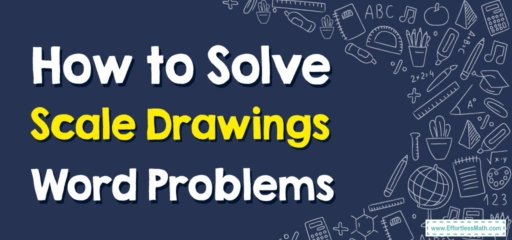# How to Solve Scale Drawings Word Problems

Scale drawings word problems involve the application of proportion and scale in real-life scenarios. These problems require a solid understanding of how to interpret and work with scale drawings, as well as the ability to set up and solve equations. In this article, we will guide you through the step-by-step process of solving scale drawings word problems. Whether you are a student studying for a math exam or an individual looking to enhance your problem-solving skills, this article will help you master the art of solving scale drawings word problems.## A Step-by-step Guide to Solving Scale Drawings Word Problems

Let’s break it down step by step:

### Step 1: Understand the Problem

The first step is to carefully read the problem and make sure you understand what you’re being asked to find. You need to identify the actual size of the object or distance and the scale used in the drawing. This is typically represented as a ratio.

### Step 2: Identify the Scale

The scale should be given in the problem. It might be something like $$1:100$$, which means that $$1$$ unit on the drawing corresponds to $$100$$ of the same units in real life. If the scale isn’t given directly, you may have to calculate it from information in the problem.

### Step 3: Set Up a Proportion

Once you’ve identified the scale, you can set up a proportion to solve for the unknown. A proportion is an equation that shows that two ratios are equivalent.

For example, if a $$1$$-inch drawing represents $$4$$ feet of a building, and you have a $$3$$-inch drawing, your proportion might look like this:

$$\frac{1 in}{4 ft}=\frac{3 in}{X ft}$$

### Step 4: Solve for the Unknown

Next, you solve the proportion for the unknown variable. To do this, you can cross-multiply and solve for the variable.

Using the above example:

$$1 in \times X ft = 3 in \times 4 ft$$

$$X = \frac{(3 in \times 4 ft)}{1 in}= 12 ft$$

So, a $$3$$-inch drawing of the building would represent a $$12$$-foot actual height.

Finally, always check your answer to make sure it makes sense in the context of the problem.

In our example, does it make sense that the building is $$12$$ feet tall if the $$3$$-inch drawing is scaled up? Since our scale was $$1$$ inch for every $$4$$ feet, and we had a $$3$$-inch drawing, it indeed makes sense that the actual height is $$3$$ times the $$4$$ feet, which is $$12$$ feet.

Remember, practice makes perfect. The more problems you work through, the better you’ll get at solving these types of problems.

### What people say about "How to Solve Scale Drawings Word Problems - Effortless Math: We Help Students Learn to LOVE Mathematics"?

No one replied yet.

X
30% OFF

Limited time only!

Save Over 30%

SAVE $5 It was$16.99 now it is \$11.99